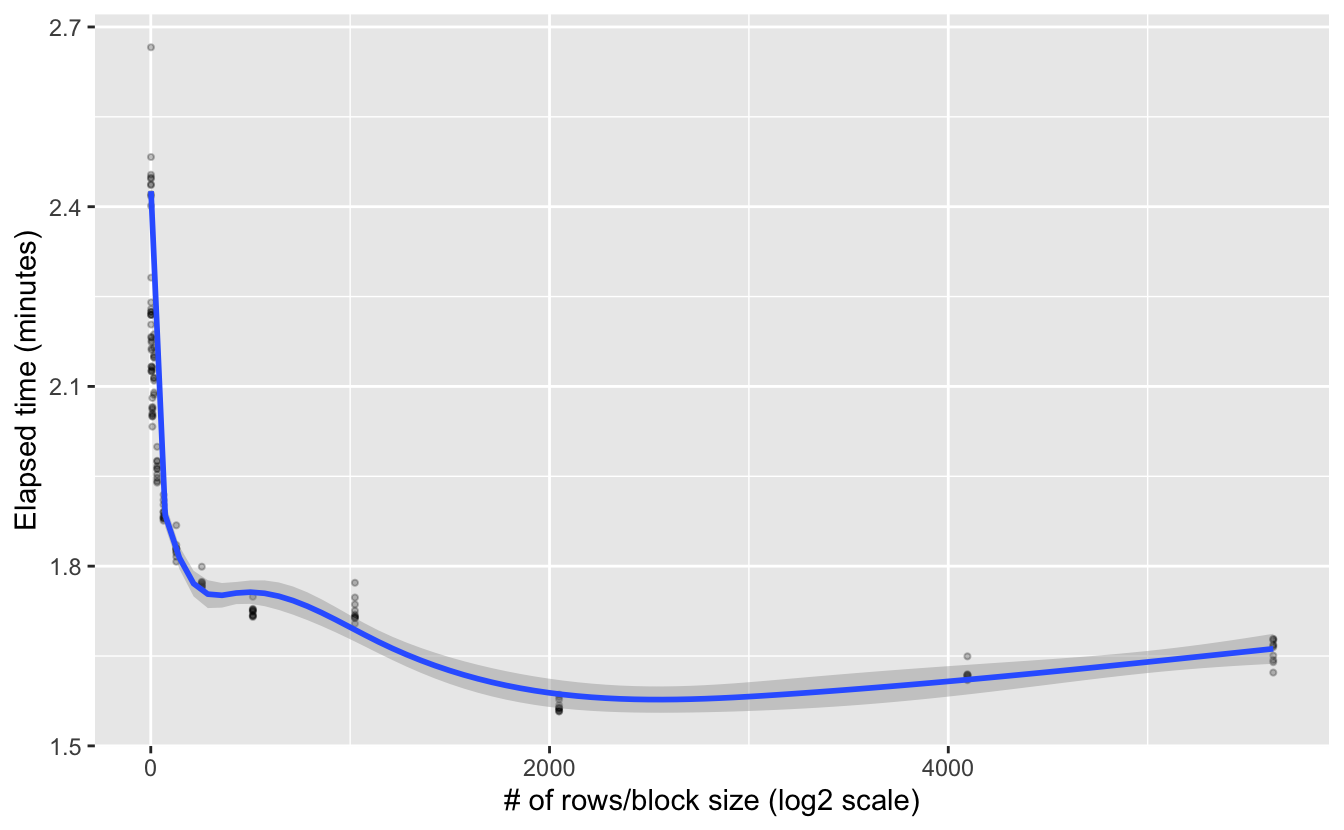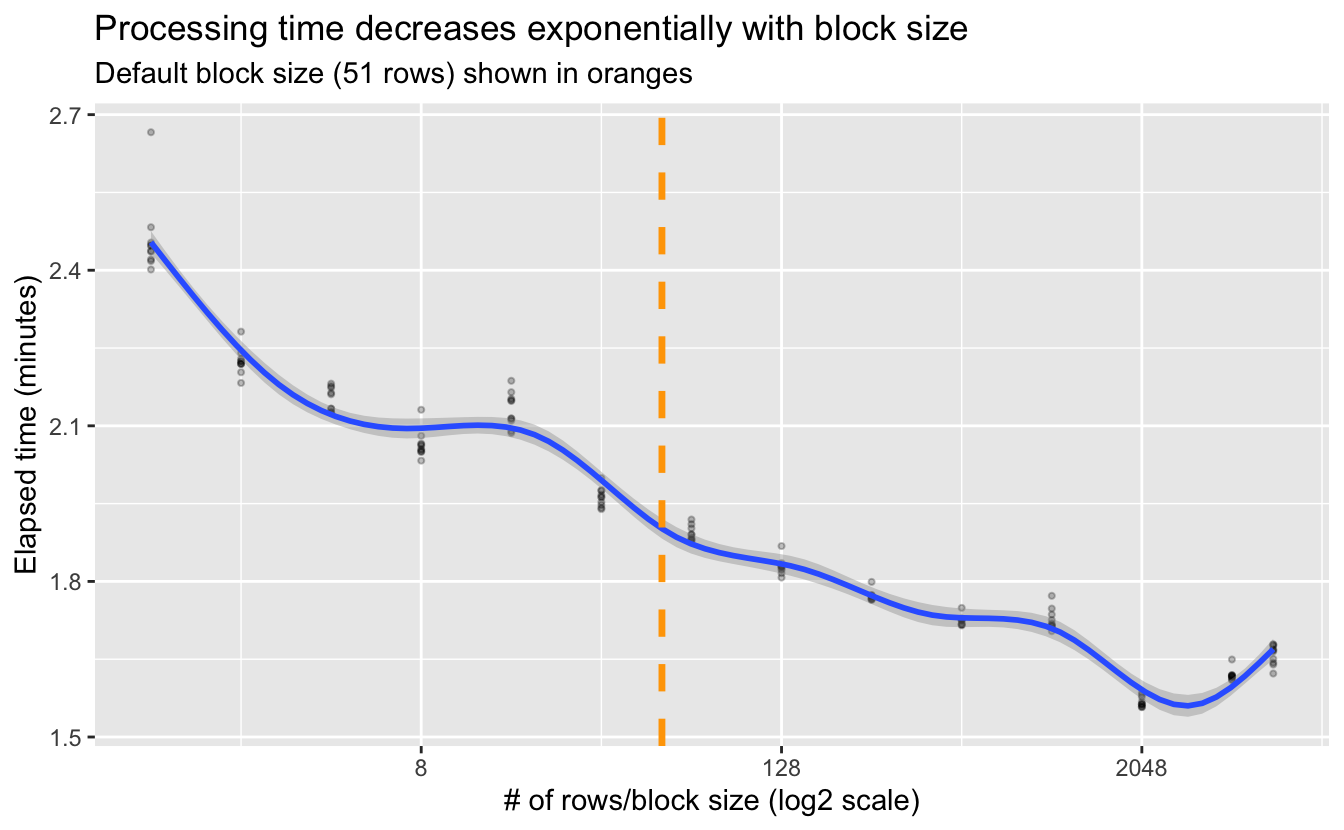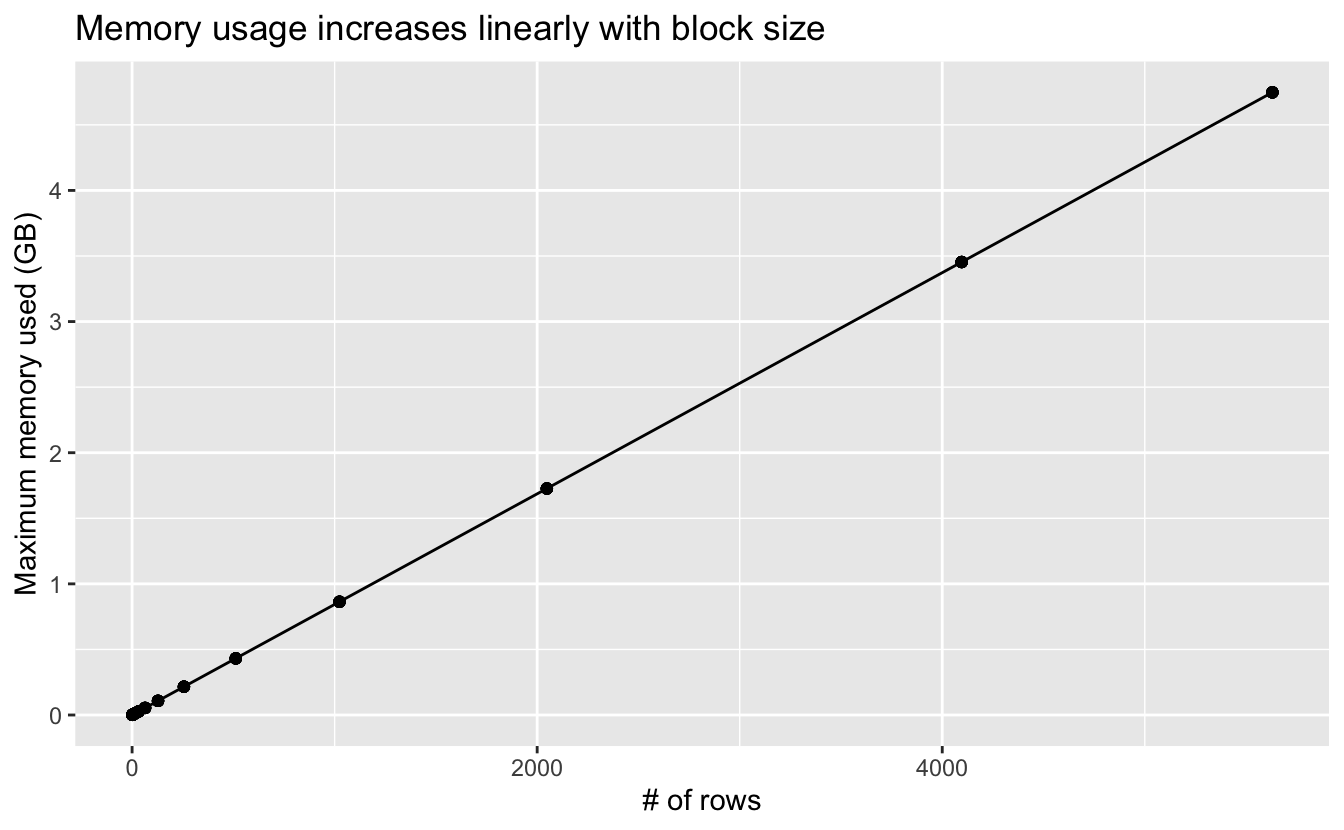# Efficient Block Processing of Rasters in R

In my previous post, I tried to understand how raster package functions like calc() process raster data. The package does a good job of abstracting a lot of this detail away from users, data will be processed either on disk or in memory based on criteria related to the size of the raster, the available resources, and user-defined parameters. In addition, if processing is done on disk, the raster will be processed in blocks, the size of which is user controlled. In most cases, raster makes sensible choices and the whole process works seamlessly for the user. However, I’ve found that, for large raster datasets, the specific details of how a raster is processed in blocks can make a big difference in terms of computational efficient. Furthermore, with raster it’s hard to know exactly what’s going on behind the scenes and the parameters that we can tune through rasterOptions() don’t give me as much direct control over the processing as I’d like. In this post, I’m going to try taking lower level control of raster processing and explore how choices about block size impact processing speed.

To demonstrate all this, I’m going to use some real data from the eBird Status & Trends; this is a dataset that I work with on a daily basis in my job. The below code downloads weekly relative abundance for Wood Thrush at 3 km resolution across the entire western hemisphere, subsets the annual data to just the weeks of the breeding season (May 24 - September 7), then saves the results as a GeoTIFF.

library(ebirdst)
library(raster)
library(tictoc)
library(profmem)
library(tidyverse)
abd <- get_species_path("woothr") %>%
# subset to the breeding season
dts <- parse_raster_dates(abd)
r <- abd[[which(dts >= as.Date("2018-05-24") & dts <= as.Date("2018-09-07"))]]
r <- writeRaster(r, "data/woothr.tif")

This is a big dataset, almost 40 million cells and 16 layers, which works out to be just under 5 GB when read into memory, assuming 8 bytes per cell.

print(r)
#> class      : RasterStack
#> dimensions : 5630, 7074, 39826620, 16  (nrow, ncol, ncell, nlayers)
#> resolution : 2963, 2963  (x, y)
#> extent     : -2e+07, 943785, -6673060, 1e+07  (xmin, xmax, ymin, ymax)
#> crs        : +proj=sinu +lon_0=0 +x_0=0 +y_0=0 +a=6371007.181 +b=6371007.181 +units=m +no_defs
#> names      : woothr.1, woothr.2, woothr.3, woothr.4, woothr.5, woothr.6, woothr.7, woothr.8, woothr.9, woothr.10, woothr.11, woothr.12, woothr.13, woothr.14, woothr.15, ...
#> min values :        0,        0,        0,        0,        0,        0,        0,        0,        0,         0,         0,         0,         0,         0,         0, ...
#> max values :     4.86,     4.76,     4.59,     4.94,     5.68,     5.59,     5.32,     4.98,     4.62,      3.75,      2.84,      2.30,      2.36,      2.57,      2.93, ...
# estimate size in gb
8 * ncell(r) * nlayers(r) / 2^30
#>  4.75

What I want to do with this dataset, is simply average across the weeks to produce a single layer of breeding season abundance, which is what we use to generate the seasonal maps on the Status and Trends website. As I showed in the previous post, that can be done with calc():

r_mean <- calc(r, mean, na.rm = TRUE)

This took about 2 minutes to run on my laptop and, given the size, it must have been processed on disk in blocks rather than in memory. Recall that the block size is the number of rows that are read in and processed at a time. The catch is that it’s not clear what block size was used and if the automatically chosen block size was optimal. You can use rasterOptions() to set chunksize in bytes, which indirectly controls the number of rows per block. Throughout this post, I’ll use “block size” to refer to the number of rows per block and “chunk size” to refer to the user-defined maximum number of bytes available for each block. If you consult the source code for calc() it’s possible to determine that this function splits rasters into blocks using blockSize() with n = 2 * (nlayers(r) + 1), then you’d have to look at the source code for blockSize() to determine that this is converted to the number of rows per blocks with floor(chunksize / (8 * n * ncol(r))). So, working through all this with the default chunk size used by raster ($$10^8$$), we get:

n <- 2 * (nlayers(r) + 1)
1e8 / (8 * n * ncol(r))
#>  52

In most cases, you’re probably best to just set chunksize based on your system’s resources and leave the block size choice to raster, but for sake of optimizing the processing of the Status & Trends data, I want to know exactly what block size is being used and, ideally, be able to control the block size directly rather than via the chunk size. With this is mind, I forked the raster GitHub repo and made some modifications.

## Modifying the raster package

After forking the raster package repository I made the following changes:

1. calc() now displays the block size (i.e. number of rows) being used.
2. calc(return_blocks = TRUE) will, instead of processing the raster object, just return the blocks that would have been used.
3. Added a new function, summarize_raster(), that’s a simplified version of calc(). It always process files on disk, will only calculate means and sums rather than a generic input function, always uses na.rm = TRUE, and it takes either a chunksize argument (in units of available GB of RAM) or an n_rows argument that directly controls the number of rows per block.

If you’re interested, the source code for the summarize_raster() function is here. It removes much of the functionality of calc() but this comes with the benefit of being easier to figure out what’s going on inside the function. If you want to install this version of the raster package you could do so with remotes::install_github("mstrimas/raster"), but beware I’ve modified the original package in a very sloppy way and you probably don’t want to overwrite the real raster package with my hacked version.

## So, what block size is being used?

tic()
r_mean_calc <- calc(r, mean, na.rm = TRUE)
#> Using 111 blocks of 51 rows.
toc()
#> 127.619 sec elapsed

In this case, with the default chunk size of $$10^8 \approx 0.1 GB$$, calc() processes this raster in 111 blocks of 51 rows each. We can use my custom summarize_raster() function to do the same thing, but specify block size explicitly.

tic()
r_mean_summ <- summarize_raster(r, "mean", n_rows = 51)
#> Using 111 blocks of 51 rows.
toc()
#> 125.852 sec elapsed

Turns out summarize_raster() is slightly faster because I’ve slimmed it down and removed some additional checks and functionality. We can confirm that the two approaches produce the same results.

cellStats(abs(r_mean_calc - r_mean_summ), max)
#>  0

## Run time vs. block size

Ok, now everything’s in place, and we can examine how block size impacts processing time. Recall that block size is the number of rows that get read in, summarized, and output at each iteration of the processing. Let’s estimate run time for a range of blocks sizes, from 1 row to 5,630 rows, equivalent to processing the whole raster in memory. Note that I’m running this on my laptop, which has 16 GB of RAM, so I have more than enough resources to process this raster in memory, but I’m choosing to process it on disk for demonstration purposes. Since there could be variation in run time, I’ll summarize the raster 10 times for each block size.

# choose the values of n_rows to try
n_rows <- 2^seq(0, ceiling(log2(nrow(r))))
n_rows[length(n_rows)] <- nrow(r)

# set up 10 repetitions of each n_rows value
nrow_grid <- expand_grid(rep = 1:10, n_rows = n_rows)

# summarize raster using each value of n_rows
summarize_nrows <- function(x) {
# memory usage
m <- profmem({
# timer
t <- system.time({
r_mean <- summarize_raster(r, "mean", n_rows = x)
})
})
return(tibble(elapsed = t["elapsed"], max_mem = max(m\$bytes, na.rm = TRUE)))
}
time_mem <- nrow_grid %>%
mutate(results = map(n_rows, summarize_nrows)) %>%
unnest(cols = results)

Now let’s plot the elapsed time vs. block size curve. I’ll show both the raw data, consisting of 10 for each block size, and a smoothed function.

ggplot(time_mem) +
aes(x = n_rows, y = elapsed / 60) +
geom_point(alpha = 0.25, size = 0.75) +
geom_smooth(method = "gam") +
labs(x = "# of rows/block size (log2 scale)",
y = "Elapsed time (minutes)")Interesting, it looks like there’s an exponential decrease in processing time as block size increases. This suggests a log-transformed x-axis would be worthwhile. I’m also going to show the default block size explicitly on the plot.

ggplot(time_mem) +
aes(x = n_rows, y = elapsed / 60) +
geom_point(alpha = 0.25, size = 0.75) +
geom_smooth(method = "gam") +
geom_vline(xintercept = 51,
color = "orange",
size = 1.2,
linetype = "dashed") +
scale_x_continuous(trans = "log2") +
labs(x = "# of rows/block size (log2 scale)",
y = "Elapsed time (minutes)",
title = "Processing time decreases exponentially with block size",
subtitle = "Default block size (51 rows) shown in oranges")Looks like the decrease is exponential. The default block size using chunksize = 1e8 does a pretty good job, but it’s not giving us the most efficient processing given the memory resources available. This shouldn’t be too surprising since $$10^8$$ bytes is quite small, so would should really be picking something more appropriate for our system’s available RAM. All this said, it’s worth noting these are fairly modest efficiency gains, but they can add up if you’re dealing with a large number of big raster files.

I also estimated the maximum memory usage during the summarization, so let’s take a look at that.

ggplot(time_mem) +
aes(x = n_rows, y = max_mem / 2^30) +
geom_line() +
geom_point() +
labs(x = "# of rows", y = "Maximum memory used (GB)",
title = "Memory usage increases linearly with block size")This is as expected: large blocks mean more data is being processed at once, which leads to higher memory usage.

The takeaway from all this is so obvious it’s almost embarrassing: if you want to process large rasters efficiently, increase the chunksize option so the raster package will process files in larger blocks and take advantage of the RAM you have available. Despite it being obvious, I suspect most people are like me and blindly go with the default raster options, which is fine in many cases, but becomes problematic for large rasters.##### Matt Strimas-Mackey
###### Data Scientist

I am a data scientist at the Cornell Lab of Ornithology using data from the citizen science project eBird to inform bird research and conservation.